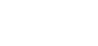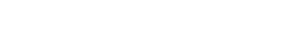How to Add and Subtract Matrices

A matrix (plural matrices) is just an array or table of numbers. What would it mean to add two tables together? Well you’re about to find out! In this short article, we’ll discuss how to add and subtract matrices.Image by Andrey Popov

What Is a Matrix?

First, let’s talk about what a matrix is.

A matrix is a rectangular array of numbers.

Each horizontal line of numbers in a matrix is a row. Each vertical line is a column. We often call the individual numbers the entries of the matrix.

If there are m rows and n columns in the array, then we say that the dimensions of the matrix are m × n. This will become important because only matrices having the same dimensions can be added or subtracted.This matrix has 2 rows and 3 columns, so its dimensions are 2 × 3.

How to Add and Subtract Matrices

There are two essential rules for adding matrices together.

1. You can only add two matrices that have the same exact dimensions. So, you can add two 3 × 4 matrices together. But you can’t add a 3 × 4 matrix to a 4 × 3 matrix. (The order of the dimensions matter too!)
2. Add corresponding entries. That is, the upper-left entry of the first matrix has to be added to the upper-left entry of the second matrix, and so on.

To subtract two matrices, AB, follow these steps:

1. Change the operation to an addition, A + (-B) by converting every entry of B to its opposite (flip signs).
2. Add the matrices, using the method above.

(You could instead just subtract the corresponding entries to compute AB, but then you’d have to keep track of subtracting negative amounts sometimes. I just find it easier to flip every sign first and then add. It amounts to the same thing algebraically.)

Examples

Consider the following three matrices:Find:

a) A + B

b) A + C

c) BA

d) CC

Solutions

a) First check the dimensions. Both A and B have two rows and two columns. Since they are both 2 × 2 matrices, they can be added together. Remember to add corresponding entries.b) This time, when we check dimensions, we find a mismatch. A is 2 × 2, but C is 3 × 2. Therefore, the sum does not exist. In other words, there’s no answer.

c) Just as in part a, both matrices have the same dimensions. So we’re allowed to subtract them. Don’t forget to flip the signs of the second one!d) Obviously C has the same dimensions as itself, so the rules say that you may subtract.

Because all of the entries are the same, when you subtract CC you end up with zero (0) in every spot.(By the way, any matrix having nothing but 0 in every entry is called a zero or null matrix.)

Author

•Shaun earned his Ph. D. in mathematics from The Ohio State University in 2008 (Go Bucks!!). He received his BA in Mathematics with a minor in computer science from Oberlin College in 2002. In addition, Shaun earned a B. Mus. from the Oberlin Conservatory in the same year, with a major in music composition. Shaun still loves music -- almost as much as math! -- and he (thinks he) can play piano, guitar, and bass. Shaun has taught and tutored students in mathematics for about a decade, and hopes his experience can help you to succeed!